# 653 and Level 4

When the last two digits of 653 are divided by 4, we get a remainder of 1 so there is an alternate way to tell if 653 is a prime number by first determining if it is the sum of any two perfect squares:

653 – 1 – 3 – 5 – 7 – 9 – 11 – 13 – 15 – 17 – 19 – 21 – 23 – 25 = 484 which is 22².

653 – 22² = 169 which is 13².

Thus 22² + 13² = 653, and 653 is the sum of two perfect squares.

From that mathematical statement we observe the following:

• 22 and 13 have no common prime factors, and 653 is odd.
• 653 is the hypotenuse of the primitive Pythagorean triple 315-572-653 which was calculated using 22² – 13², 2(22)(13), 22² + 13².
• The only primitive Pythagorean triple hypotenuses less than √653 are 5, 13, and 17.
• 653 obviously is not divisible by 5 or 13.
• If 653 is not divisible by 17, it is a prime number. 653 is not divisible by 17.

Print the puzzles or type the solution on this excel file: 12 Factors 2015-10-19

——————————————————————

• 653 is a prime number.
• Prime factorization: 653 is prime.
• The exponent of prime number 653 is 1. Adding 1 to that exponent we get (1 + 1) = 2. Therefore 653 has exactly 2 factors.
• Factors of 653: 1, 653
• Factor pairs: 653 = 1 x 653
• 653 has no square factors that allow its square root to be simplified. √653 ≈ 25.55386.How do we know that 653 is a prime number? If 653 were not a prime number, then it would be divisible by at least one prime number less than or equal to √653 ≈ 25.6. Since 653 cannot be divided evenly by 2, 3, 5, 7, 11, 13, 17, 19, or 23, we know that 653 is a prime number.

We also know that 653 is a prime number from the observations made at the beginning of this post.

—————————————————————————————————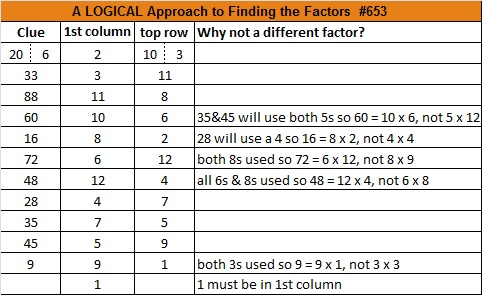# 651 and Level 2

21 x 31 = 651. Both of those factors are 5 away from their average, 26, so 651 is 25 less than 26² or 676.

The numbers in each of 651’s four factor pairs are odd, and the average of each and the distance each is from that average are both whole numbers. That means that 651 can be expressed as the difference of two squares four different ways. In this particular case the averages and distances generate four primitive Pythagorean triples with 651 as one of the legs:

• 26² – 5² = 651; primitive triple 260-651-701
• 50² – 43² = 651; primitive triple 651-4300-4349
• 110² – 107² = 651; primitive triple 651-23540-23549
• 326² – 325² = 651; primitive triple 651-211900-211901

651 is also a  pentagonal number.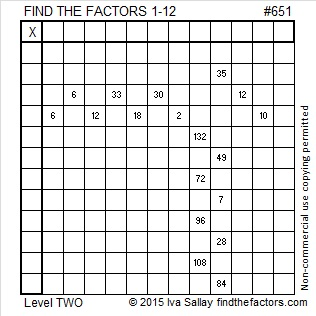Print the puzzles or type the solution on this excel file: 12 Factors 2015-10-19

—————————————————————————————————

• 651 is a composite number.
• Prime factorization: 651 = 3 x 7 x 31
• The exponents in the prime factorization are 1, 1, and 1. Adding one to each and multiplying we get (1 + 1)(1 + 1)(1 + 1) = 2 x 2 x 2 = 8. Therefore 651 has exactly 8 factors.
• Factors of 651: 1, 3, 7, 21, 31, 93, 217, 651
• Factor pairs: 651 = 1 x 651, 3 x 217, 7 x 93, or 21 x 31
• 651 has no square factors that allow its square root to be simplified. √651 ≈ 25.5147.—————————————————————————————————# I can tell that 613 is a prime number just by looking at it!

613 is the 18th centered square number because 17 and 18 are consecutive numbers and 18² + 17² = 613.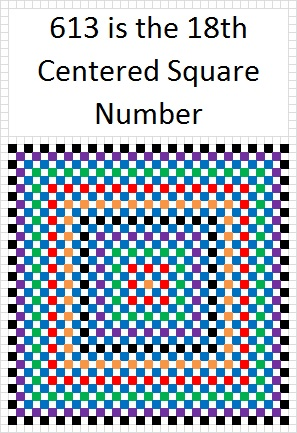613 is the hypotenuse of the primitive Pythagorean triple 35-612-613, which was calculated using (18^2) – (17^2), 2 x 17 x 18, and (18^2) + (17^2).

Knowing that (18^2) + (17^2) = 613, I can tell that 613 is a prime number just by looking at it! Here’s what I see:

• 613 obviously is not divisible by 5.
• It is almost as obvious that 613 is not divisible by 13.
• 18^2 is not divisible by 17 so (18^2) + (17^2) is not divisible by 17. Thus 613 is not divisible by 17.
• Since 613 is not divisible by 5, 13, or 17, it is a prime number.

“What?” you say. What about all the other possible prime factors less than 24.8, the approximate value of √613? Don’t we need to TRY to divide 613 by 2, 3, 7, 11, 19, and 23?

No, we don’t. Those numbers don’t matter at all. Since 17^2 + 18^2 = 613, and because 17 and 18 have NO common prime factors and only one of them is odd, I already know that none of those numbers will divide into 613. Let me show you what I mean.

Look at this chart of ALL the primitive Pythagorean triple hypotenuses less than 1000:The numbers in red are all prime numbers and appear on the chart only one time. Every prime number less than 1000 that can be written in the form 4N + 1 appears somewhere on the chart. 613 can be found at the intersection of the column under 17 and the row labeled 18.

The black numbers on the colorful squares are composite numbers and many of them appear on the chart above more than one time. There is something very interesting about the prime factors of these composite numbers. Every single one of those factors is also the hypotenuse of a primitive Pythagorean triple! Also notice that at least one of those factors is less than or equal to the square root of the composite number. Here is a chart of those composite numbers, how many times they appear on the chart above, and their prime factorizations:Also notice that if you divide any of the above composite numbers OR their factors by 4, the remainder is always 1.

I have demonstrated that the following is true for any integer less than 1000. I wasn’t sure if it had been proven for integers in general so I asked Gordan Savin, a professor at the University of Utah, who teaches number theory. He informed me that it has been proven and that it follows from the uniqueness of factorization of Gaussian integers.

In conclusion, if an integer can be written as the sum of two squares, one odd and one even, and the numbers being squared have no common prime factors, then ALL the factors of that odd integer will be hypotenuses of primitive Pythagorean triples. If the integer is a composite number, at least one of those primitive hypotenuses will be less than or equal to the square root of that integer.

Here’s the same thing in more standard mathematical language:

If a natural number of the form 4N + 1 can be written as the sum of two squares (a^2)+ (b^2) and if a and b have no common prime factors, then ALL the factors of 4N + 1 will be of the form 4n + 1. If 4N + 1 is a composite number, there will exist at least one prime factor, 4n + 1 ≤  √(4N + 1). If no such factor exists, then 4N + 1 is a prime number.

• 613 is a prime number.
• Prime factorization: 613 is prime.
• The exponent of prime number 613 is 1. Adding 1 to that exponent we get (1 + 1) = 2. Therefore 613 has exactly 2 factors.
• Factors of 613: 1, 613
• Factor pairs: 613 = 1 x 613
• 613 has no square factors that allow its square root to be simplified. √613 ≈ 24.7588How do we know that 613 is a prime number? If 613 were not a prime number, then it would be divisible by at least one prime number less than or equal to √613 ≈ 24.8. Since 613 cannot be divided evenly by 2, 3, 5, 7, 11, 13, 17, 19, or 23, we know that 613 is a prime number. But as I explained above since (18^2) + (17^2) = 613, and consecutive integers 18 and 17 have no common prime factors, it is only necessary to check to see if 613 is divisible by 5, 13, or 17. Since it isn’t divisible by any of those three numbers, 613 is a prime number.

# 601 The Other Side of a Twin Prime

601 and 599 are twin primes because there is only one number between them. That number happens to be 600, a number with 24 factors.

Twin primes certainly are not identical twins. That solitary number between them and the fact that they are both primes is usually all they have in common.

Other famous twin primes are 11 and 13, 17 and 19, 29 and 31, and 41 and 43.

3, 5, 7 is the only prime triplet that consists of three consecutive odd numbers because 3 is the only prime number divisible by 3, and all sets of three consecutive odd numbers must have at least one number in the set that is divisible by 3. Other than 2, 3, 5, all other so-called prime triplets come from four consecutive odd numbers. Three of the four numbers will be prime while either the second or the third number will not be.

Twin primes like 599 and 601 can be prime factors. That is something 600 can never do.

For example, 599 x 601 = 359, 999 which is 600² – 1. They are its prime factors. 600 is a factor of an infinite amount of numbers, but it is not a prime factor of any number.

What are some ways twin primes are different from each other? I’ll use twin primes 599 and 601 as examples.

When each prime in a twin prime is divided by 4, the remainder will be 1 for one of them and 3 for the other. The one that has a remainder of 1 will be the sum of two square numbers and will also be the hypotenuse of a primitive Pythagorean triple. The one with a remainder of 3 will never be either of those things. In the triple and twin primes listed above 5, 13, 17, 29, 41, and 601 have a remainder of 1 when divided by 4.

601 = 24² + 5², and is the hypotenuse of the primitive Pythagorean triple 480, 551, 601 while 599 doesn’t do either of those things.

599 is the smallest number whose digits add up to 23. If you add up 601’s digits, you’ll get 7. Figure out how many numbers less than 601 have digits that add up to 7. It’s a lot.

601 has accomplished some other impressive things:1 + 5 + 10 + 15 + 20 + 25 + 30 + 35 + 40 + 45 + 50 + 55 + 60 + 65 + 70 + 75 = 601 which makes 601 the 16th centered pentagonal number

Because it is a centered pentagonal number, 601 is one more than five times the 15th triangular number.

The factors of numbers from 601 to 607 form a symmetrical shape.I gave the factoring information for 599 previously. Here is the information for 601:

• 601 is a prime number.
• Prime factorization: 601 is prime.
• The exponent of prime number 601 is 1. Adding 1 to that exponent we get (1 + 1) = 2. Therefore 601 has exactly 2 factors.
• Factors of 601: 1, 601
• Factor pairs: 601 = 1 x 601
• 601 has no square factors that allow its square root to be simplified. √601 ≈ 24.5153How do we know that 601 is a prime number? If 601 were not a prime number, then it would be divisible by at least one prime number less than or equal to √601 ≈ 24.5. Since 601 cannot be divided evenly by 2, 3, 5, 7, 11, 13, 17, 19, or 23, we know that 601 is a prime number.

# 577 and Level 6

24² + 1 = 577 means 577 is the hypotenuse of a primitive Pythagorean triple which happens to be 48-575-577.Print the puzzles or type the solution on this excel file: 10 Factors 2015-08-03

—————————————————————————————————

• 577 is a prime number.
• Prime factorization: 577 is prime.
• The exponent of prime number 577 is 1. Adding 1 to that exponent we get (1 + 1) = 2. Therefore 577 has exactly 2 factors.
• Factors of 577: 1, 577
• Factor pairs: 577 = 1 x 577
• 577 has no square factors that allow its square root to be simplified. √577 ≈ 24.020824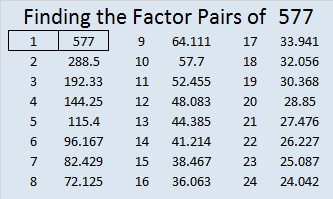How do we know that 577 is a prime number? If 577 were not a prime number, then it would be divisible by at least one prime number less than or equal to √577 ≈ 24.02. Since 577 cannot be divided evenly by 2, 3, 5, 7, 11, 13, 17, 19, or 23, we know that 577 is a prime number.

—————————————————————————————————# 541 and Level 5

541 = 21² + 10² = 441 + 100

541 is the hypotenuse of the primitive Pythagorean triple 341-420-541

And finally, after the longest string of composite numbers so far, 541 is quite notably a prime number. In fact, it is the 100th prime number.Print the puzzles or type the solution on this excel file: 12 Factors 2015-06-29

—————————————————————————————————

• 541 is a prime number.
• Prime factorization: 541 is prime.
• The exponent of prime number 541 is 1. Adding 1 to that exponent we get (1 + 1) = 2. Therefore 541 has exactly 2 factors.
• Factors of 541: 1, 541
• Factor pairs: 541 = 1 x 541
• 541 has no square factors that allow its square root to be simplified. √541 ≈ 23.25940 66 99 22 601 44How do we know that 541 is a prime number? If 541 were not a prime number, then it would be divisible by at least one prime number less than or equal to √541 ≈ 23.3. Since 541 cannot be divided evenly by 2, 3, 5, 7, 11, 13, 17, 19, or 23, we know that 541 is a prime number.

—————————————————————————————————# 461 and Level 3

461 = 19² + 10², and it is the hypotenuse in this primitive Pythagorean triple: 261-380-461.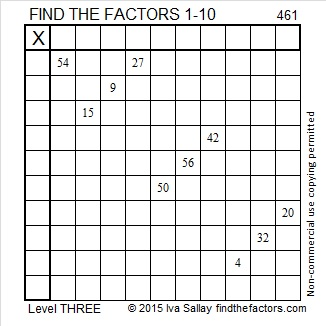Print the puzzles or type the solution on this excel file:  10 Factors 2015-04-13

—————————————————————————————————

• 461 is a prime number.
• Prime factorization: 461 is prime.
• The exponent of prime number 461 is 1. Adding 1 to that exponent we get (1 + 1) = 2. Therefore 461 has exactly 2 factors.
• Factors of 461: 1, 461
• Factor pairs: 461 = 1 x 461
• 461 has no square factors that allow its square root to be simplified. √461 ≈ 21.4709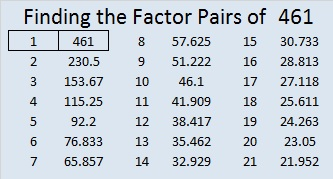How do we know that 461 is a prime number? If 461 were not a prime number, then it would be divisible by at least one prime number less than or equal to √461 ≈ 21.4709. Since 461 cannot be divided evenly by 2, 3, 5, 7, 11, 13, 17, or 19, we know that 461 is a prime number.

—————————————————————————————————

A Logical Approach to solve a FIND THE FACTORS puzzle: Find the column or row with two clues and find their common factor. Write the corresponding factors in the factor column (1st column) and factor row (top row).  Because this is a level three puzzle, you have now written a factor at the top of the factor column. Continue to work from the top of the factor column to the bottom, finding factors and filling in the factor column and the factor row one cell at a time as you go.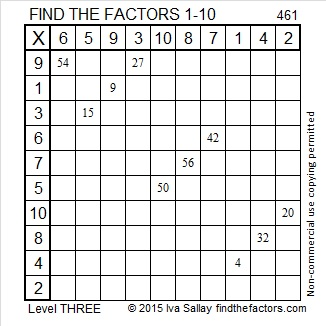# 457 A Pythagorean Triple Logic Puzzle

457 = 4² + 21², and it is the hypotenuse of the primitive Pythagorean triple 168-425-457. Also, 457 is the sum of some consecutive prime numbers. One of my readers posted those primes in the comments.

A long time ago I decided that Pythagorean triples could make a great logic puzzle, so I created one. You can see it directly underneath the following directions:

This puzzle is NOT drawn to scale. Angles that are marked as right angles are 90 degrees, but any angle that looks like a 45 degree angle, isn’t 45 degrees. Lines that look parallel are NOT parallel. Shorter looking line segments may actually be longer than longer looking line segments. Most rules of geometry do not apply here: in fact non-adjacent triangles in the drawing might actually overlap.

No geometry is needed to solve this puzzle. All that is needed is the table of Pythagorean triples under the puzzle. The puzzle only uses triples in which each leg and each hypotenuse is less than 100 units long. The puzzle has only one solution.

If any of these directions are not clear, let me know in the comments. I will NOT be publishing the solution to this puzzle, but I will allow anyone who desires to put any or all of the missing values in the comments. Also, the comments will help me determine if I should publish another puzzle like this one.

Good Luck!Print the puzzles or type the solution on this excel file:  10 Factors 2015-04-13

—————————————————————————————————

• 457 is a prime number.
• Prime factorization: 457 is prime.
• The exponent of prime number 457 is 1. Adding 1 to that exponent we get (1 + 1) = 2. Therefore 457 has exactly 2 factors.
• Factors of 457: 1, 457
• Factor pairs: 457 = 1 x 457
• 457 has no square factors that allow its square root to be simplified. √457 ≈ 21.3776How do we know that 457 is a prime number? If 457 were not a prime number, then it would be divisible by at least one prime number less than or equal to √457 ≈ 21.3776. Since 457 cannot be divided evenly by 2, 3, 5, 7, 11, 13, 17, or 19, we know that 457 is a prime number.

# 396 What Pythagorean Triple Comes Next?

396 is a multiple of 4, but not of 8, so just like 12, 20, 28, and 36, it is a leg of a primitive Pythagorean triple that is included in this infinite sequence of primitive triples (12513), (202129), (284553), (367785) . . . , which I’ve illustrated below:Because they are Pythagorean triples, we know that 12² + 5² = 13², 20² + 21² = 29², 28² + 45² = 53², 36² + 77² = 85², and so forth.

What Pythagorean Triple Comes Next? Try to figure it out yourself, then scroll down a little bit to see if you are correct. In the meantime, let me tell you a little bit about the number 396:

• 396 is a composite number.
• Prime factorization: 396 = 2 x 2 x 3 x 3 x 11, which can be written 396 = (2^2) x (3^2) x 11
• The exponents in the prime factorization are 2, 2 and 1. Adding one to each and multiplying we get (2 + 1)(2 + 1)(1 + 1) = 3 x 3 x 2 = 18. Therefore 396 has exactly 18 factors.
• Factors of 396: 1, 2, 3, 4, 6, 9, 11, 12, 18, 22, 33, 36, 44, 66, 99, 132, 198, 396
• Factor pairs: 396 = 1 x 396, 2 x 198, 3 x 132, 4 x 99, 6 x 66, 9 x 44, 11 x 36, 12 x 33 or 18 x 22
• Taking the factor pair with the largest square number factor, we get √396 = (√11)(√36) = 6√11 ≈ 19.8997The next primitive Pythagorean triple in the sequence can be illustrated like this:Let me tell you about five Pythagorean triples in which 396 is one of the legs:

• The answer to which Pythagorean triple comes next was (44117125), and is illustrated above. If we multiply that triple by 9, we get (396-1053-1125).
• Because 396 equals 36 x 11, another triple can be found by multiplying the previous primitive in the sequence (367785) by 11 to get (396-847-935).
• If we multiply the first triple in the sequence (12513by 33, we get (396-165-429).
• The 16th primitive triple in the sequence is (13210851093). If we multiply it by 3 we get (396-3255-3279).
• The 49th Pythagorean triple in our sequence of primitive triples above has a short leg that could be illustrated with 396 yellow squares. That primitive Pythagorean triple is (39697979805).

# 383 is in only one Pythagorean triple, and it’s primitiveA Primitive Threesome https://www.mycomicshop.com/search?TID=243401

Although it would take awhile to count to 383, it is still a relatively small number. If you scroll down, you will see that it is also a prime number. Since all odd numbers greater than one are the short legs of a primitive Pythagorean triple, 383 is also. I will explain how to find that primitive triple using the Flintstones, the primitive yet modern stone age family as an analogy. You can use this method on ANY odd number greater than one to find a primitive triple:

• 383 is like Pebbles the shortest one in the family
• If we round down (383^2)/2 or 73344.5 to 73,344, we get a much bigger number, much like Fred is much taller than Pebbles in the family
• If we round the same expression up, we get 73,345, much like Wilma is just a little taller than Fred.
• 383 – 73344 – 73345 is a primitive Pythagorean triple just as Pebbles, Fred, and Wilma are a primitive yet modern stone age family of three. Test it out: (383^2) + (73344^2) = (73345^2)

Prime numbers can be one of the legs of only one Pythagorean triple, and it will always be a primitive. Sometimes prime numbers are the hypotenuse of a primitive Pythagorean triple, but 383 isn’t. In a future post, I’ll explain how to determine if a prime number is ever the hypotenuse.

Here is 383’s factoring information:

• 383 is a prime number.
• Prime factorization: 383 is prime.
• The exponent of prime number 383 is 1. Adding 1 to that exponent we get (1 + 1) = 2. Therefore 383 has exactly 2 factors.
• Factors of 383: 1, 383
• Factor pairs: 383 = 1 x 383
• 383 has no square factors that allow its square root to be simplified. √383 ≈ 19.570How do we know that 383 is a prime number? If 383 were not a prime number, then it would be divisible by at least one prime number less than or equal to √383 ≈ 19.570. Since 383 cannot be divided evenly by 2, 3, 5, 7, 11, 13, 17, or 19, we know that 383 is a prime number.